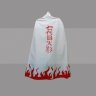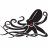# Suspicious Volume Indicator for ThinkorSwim#### BenTen

Staff
VIP
This indicator helps to identify suspicious volume.

The ratio of the current volume to the average of several previous candlesticks has been defined as standardized between 0 and 100.

When the volume line goes above 70 or below 30, we can assume that the trading volume is abnormal.### thinkScript Code

Code:
``````# Suspicious Volume
# Assembled by BenTen at useThinkScript.com

declare lower;

def Totlavol = volume;
input Ma_period = 10;
input highVol = 70;
input lowVol = 30;

def smaVol = simpleMovingAvg(Totlavol, Ma_period);
def volnow = volume;
def SVolume_index = (volnow / smaVol);
def sv_index = (100 - (100 / (1 + SVolume_index)));

plot h_vol = highVol;
plot l_vol = lowVol;

plot line = sv_index;``````

Last edited:
•jrchezz and RickAns
N

#### nashkhash

##### New member
Thanks for sharing.

•BenTen#### Birdie

##### New member
2019 Donor
I think I'll incorporate this one into my swings that I buy EOD

•BenTen
A

#### alphabeta

##### Member
This indicator helps to identify suspicious volume.

When the volume line goes above 70 or below 30, we can assume that the trading volume is abnormal.### thinkScript Code

Code:
``````# Suspicious Volume
# Assembled by BenTen at useThinkScript.com

declare lower;

def Totlavol = volume;
input Ma_period = 10;
input highVol = 70;
input lowVol = 30;

def smaVol = simpleMovingAvg(Totlavol, Ma_period);
def volnow = volume;
def SVolume_index = (volnow / smaVol);
def sv_index = (100 - (100 / (1 + SVolume_index)));

plot h_vol = highVol;
plot l_vol = lowVol;

plot line = sv_index;``````
thank you for sharing
is it possible to place alert when it goes above 70 it woluld be very helpful if possible

T

#### tomsk

##### Well-known member
VIP
thank you for sharing
is it possible to place alert when it goes above 70 it woluld be very helpful if possible

@alphabeta Yep, all you need to do is to add the following line to the end of the study and you'll be all set for that alert

Code:
``Alert(sv_index crosses above highVol, "Crosses above 70 threshold", Alert.BAR, Sound.Ring);``

•BenTen
A

#### alphabeta

##### Member
@alphabeta Yep, all you need to do is to add the following line to the end of the study and you'll be all set for that alert

Code:
``Alert(sv_index crosses above highVol, "Crosses above 70 threshold", Alert.BAR, Sound.Ring);``
thank you so much!!!!!!

W

#### Wright1

##### New member
VIP
Hey new member here!Do the 30 and 70 levels indicate buying and selling or are they just general?#### BenTen% change watchlist column based on volume and shares? Questions 0